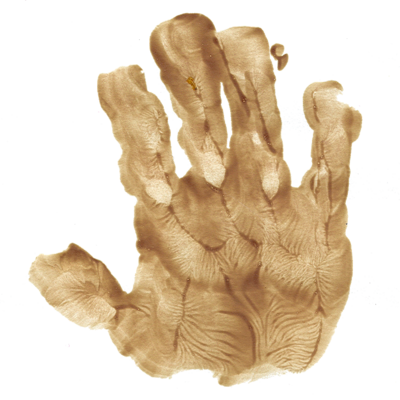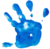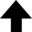Chaos: when the present determines the future, but the approximate present does not approximately determine the future.Edward Lorenz

One of the earliest indications of chaotic behavior was in an atmospheric model developed in 1960 by the meteorologist Edward Norton Lorenz; this model was detailed in "Deterministic Nonperiodic Flow" (1963). The atmosphere, in this model, was idealized in a set of 12 equations that described the motion of gas as a rotating, stratified fluid. These equations, when coded into an LGP-30 computer by Ellen Fetter and Margaret Hamilton, produced results that were difficult to visualize. Recognizing this obstacle, Lorenz decided to narrow the focus of the project by borrowing just three equations from his colleague Barry Saltzman. This simpler non-periodic system described a beaker (or cell) of water heated from below and cooled from above. The result is convection, where warm fluid at the bottom rises, then cools at the top, and finally descends as a result. Lorenz simplified the description by restricting the motion to two spatial dimensions. This phenomenon has been observed in the laboratory and is known as a Rayleigh-Bénard cell.

In the equations below, the dimensionless parameters $\sigma$, $\rho$, and $\beta$ are determined by various fluid properties, the size of the Rayleigh-Bénard cell, and the temperature difference within the cell, respectively. (In the program, $\rho$ = r and $\beta$ = b). Note that the variables $x$, $y$, and $z$ have nothing to do with actual spatial coordinates, but are instead simply convenient measures of the system state, such as convection "rate" and temperature variations within the idealized beaker. This exercise is meant to reinforce the idea that even simple sets of equations can exhibit chaotic behavior.

Need more chaos in your life? Try Hamiltonian Chaos!

Reference• Sokol, Joshua. "The Hidden Heroines of Chaos." Quanta Magazine.

Rayleigh-Bénard Convection

This program obtains a numerical solution to the Lorenz equations via a common fourth-order Runge-Kutta method.
$\displaystyle{ \frac{\mathrm{d} x}{\mathrm{d} t} = \sigma (y - x) }$ $\displaystyle{ \frac{\mathrm{d} y}{\mathrm{d} t} = x (\rho - z) - y }$ $\displaystyle{ \frac{\mathrm{d} z}{\mathrm{d} t} = x y - \beta z }$
 Parameterized fluid velocity $x$ circulating around the cell: Parameterized side-to-side temperature difference $y$: Parameterized top-to-bottom temperature difference $z$: Dimensionless parameter $\sigma$ of the system (determined by various fluid properties): Dimensionless parameter $\rho$ of the system (determined by the size of the Rayleigh-Bénard cell): Dimensionless parameter $\beta$ of the system (determined by the temperature difference within the cell): Time-step $\mathrm{d} t$ of the system: sec0.001 ≤ $\mathrm{d} t$ ≤ 0.1 sec
0%
Current data point: